# Difference between revisions of "Structured Types"

## Modelling Structured Types

The Event-B mathematical language currently does not support a syntax for the direct definition of structured types such as records or class structures. Nevertheless it is possible to model structured types using projection functions to represent the fields/attributes. For example, suppose we wish to model a record structure R with fields e and f (with type E and F respectively). Let us use the following syntax for this (not part of Event-B syntax):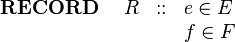$\begin{array}{lcl} \textbf{RECORD}~~~~ R &::& e\in E\\ && f \in F \end{array}$

We can model this structure in Event-B by introducing (in a context) a carrier set R and two functions e and f as constants as follows: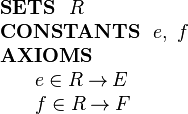$\begin{array}{l} \textbf{SETS}~~ R\\ \textbf{CONSTANTS}~~ e,~ f\\ \textbf{AXIOMS}\\ ~~~~\begin{array}{l} e \in R \tfun E\\ f \in R \tfun F\\ \end{array} \end{array}$

Now, given an element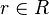$r\in R$ representing a record structure, we write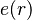$e(r)$ for the e component of r and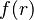$f(r)$ for the f component of r.

E and F can be any type definable in Event-B, including a type representing a record structure.

## Constructing Structured Values

Suppose we have a variable v in a machine whose type is the structure R defined above: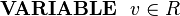$\textbf{VARIABLE}~~ v\in R$

We wish to assign a structured value to v whose e field has some value e1 and whose f field has some value f1. This can be achieved by specifying the choice of an event parameter r whose fields are constrained by appropriate guards and assigning parameter r to the machine variable v. This is shown in the following event: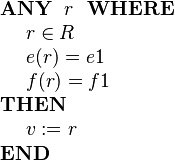$\begin{array}{l} \textbf{ANY}~~ r ~~\textbf{WHERE} \\ ~~~~ r \in R \\ ~~~~ e( r ) = e1 \\ ~~~~ f( r ) = f1 \\ \textbf{THEN} \\ ~~~~ v := r \\ \textbf{END} \end{array}$

If we only wish to update some fields and leave others changed, this needs to be done by specifying explicitly that some fields remain unchanged. This is shown in the following example where only the e field is modified: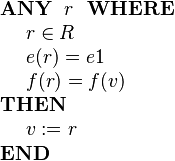$\begin{array}{l} \textbf{ANY}~~ r ~~\textbf{WHERE} \\ ~~~~ r \in R \\ ~~~~ e( r ) = e1 \\ ~~~~ f( r ) = f(v) \\ \textbf{THEN} \\ ~~~~ v := r \\ \textbf{END} \end{array}$

If we don't care about the value of some field (e.g., f), we simply omit any guard on that field as follows: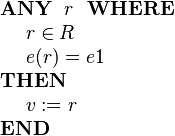$\begin{array}{l} \textbf{ANY}~~ r ~~\textbf{WHERE} \\ ~~~~ r \in R \\ ~~~~ e( r ) = e1 \\ \textbf{THEN} \\ ~~~~ v := r \\ \textbf{END} \end{array}$

Sometimes we will wish to model a set of structured elements as a machine variable, e.g.,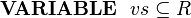$\textbf{VARIABLE}~~ vs\subseteq R$

We can add a structured element to this set using the following event: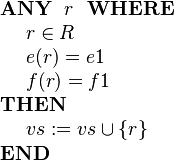$\begin{array}{l} \textbf{ANY}~~ r ~~\textbf{WHERE} \\ ~~~~ r \in R \\ ~~~~ e( r ) = e1 \\ ~~~~ f( r ) = f1 \\ \textbf{THEN} \\ ~~~~ vs := vs \cup \{r\} \\ \textbf{END} \end{array}$